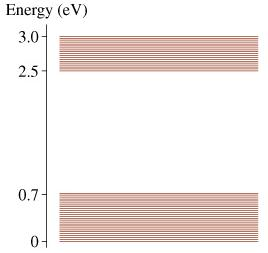# Problem: The figure above shows a molecular energy-level diagram.Part AWhat is the longest wavelength in the molecule’s absorption spectrum?= ? nmPart BWhat is the shortest wavelength in the molecule’s absorption spectrum?= ? nmPart CWhat is the longest wavelength in the molecule’s fluorescence spectrum?= ? nmPart DWhat is the shortest wavelength in the molecule’s fluorescence spectrum?= ? nm

###### FREE Expert Solution

Energy change of a molecule is expressed as:

$\overline{){\mathbf{∆}}{\mathbf{E}}{\mathbf{=}}\frac{\mathbf{h}\mathbf{c}}{\mathbf{\lambda }}}$,

93% (138 ratings)###### Problem DetailsThe figure above shows a molecular energy-level diagram.

Part A
What is the longest wavelength in the molecule’s absorption spectrum?
= ? nm

Part B
What is the shortest wavelength in the molecule’s absorption spectrum?
= ? nm

Part C
What is the longest wavelength in the molecule’s fluorescence spectrum?
= ? nm

Part D
What is the shortest wavelength in the molecule’s fluorescence spectrum?
= ? nm